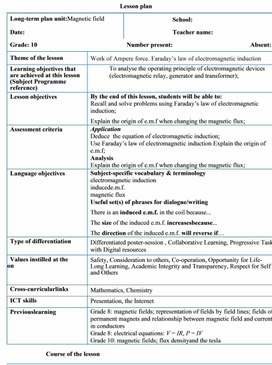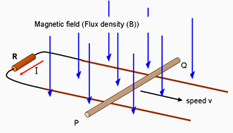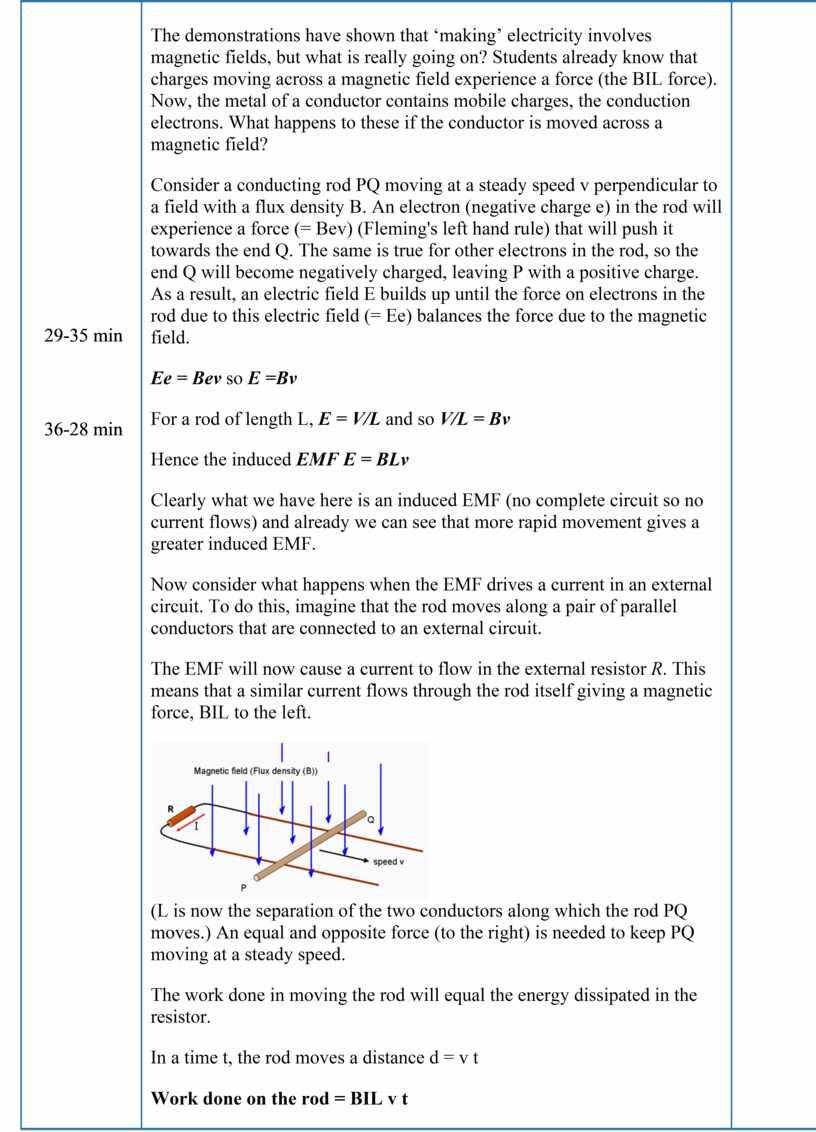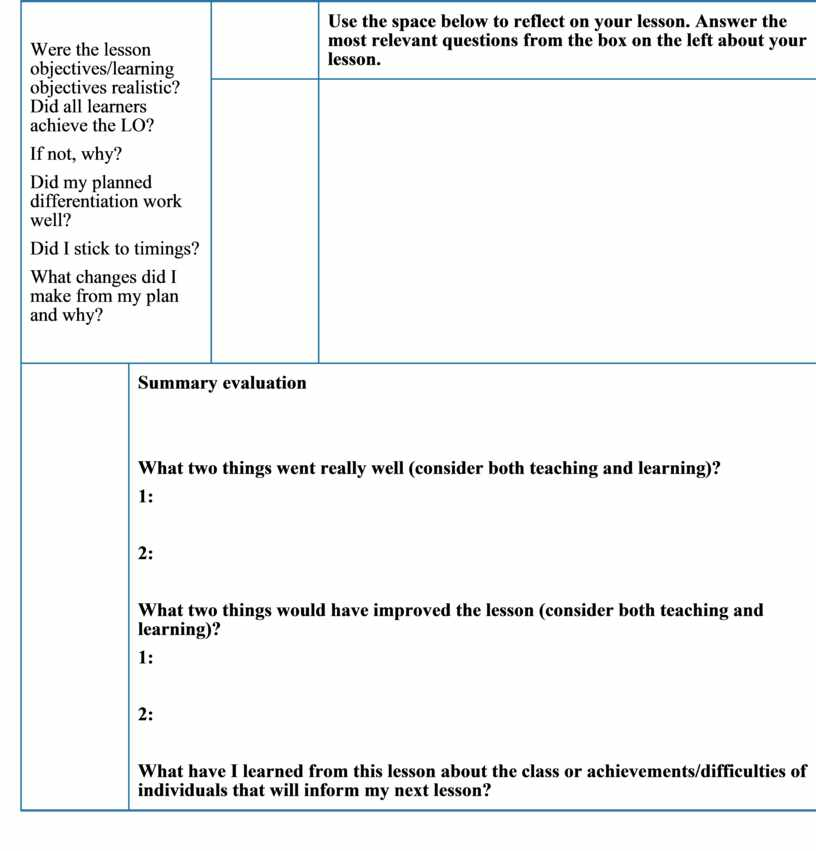2Ampere force. Faraday’s law of electromagnetic induction
Оценка 4.8

# 2Ampere force. Faraday’s law of electromagnetic induction

Оценка 4.8
docx
07.05.20202Ampere force. Faraday’s law of electromagnetic induction.docx

Lesson plan

 Long-term plan unit:Magnetic field School: Date: Teacher name: Grade: 10 Number present: Absent: Theme of the lesson Work of Ampere force. Faraday’s law of electromagnetic induction Learning objectives that are achieved at this lesson (Subject Programme reference) To analyse the operating principle of electromagnetic devices (electromagnetic relay, generator and transformer); Lesson objectives By the end of this lesson, students will be able to: Recall and solve problems using Faraday’s law of electromagnetic induction; Explain the origin of e.m.f when changing the magnetic flux; Assessment criteria Application Deduce  the equation of electromagnetic induction; Use Faraday’s law of electromagnetic induction Explain the origin of e.m.f; Analysis Explain the origin of e.m.f when changing the magnetic flux; Language objectives Subject-specific vocabulary & terminology electromagnetic induction inducede.m.f. magnetic flux Useful set(s) of phrases for dialogue/writing There is an induced e.m.f. in the coil because... The size of the induced e.m.f. increasesbecause... The direction of the induced e.m.f. will reverse if… Type of differentiation Differentiated poster-session , Collaborative Learning, Progressive Task with Digital resources Values instilled at the lesson Safety, Consideration to others, Co-operation, Opportunity for Life-Long Learning, Academic Integrity and Transparency, Respect for Self and Others Cross-curricularlinks Mathematics, Chemistry ICT skills Presentation, the Internet Previouslearning Grade 8: magnetic fields; representation of fields by field lines; fields of permanent magnets and relationship between magnetic field and current in conductors Grade 8: electrical equations: V = IR, P = IV Grade 10: magnetic fields; flux densityand the tesla Course of the lesson Planned stages of the lesson Planned activities at the lesson Resources Beginning (0-3 min)                        (4-7min) Teacher: -Introduces the topic of day and spelling out the learning outcome they will possess after the study. 1. Organizational moment to acquaint students with the ·         The theme of the lesson ·         The objectives of the lesson ·         The criteria of success for the lesson ·         The plan of events for the lesson   Discussion: How is the ‘electricity’ made? •      The previous demonstrations showed that ‘making’ electricity involves magnetic fields, but what is really going on? Middle 8-28 min                                                                                                                                              29-35 min        36-28 min The demonstrations have shown that ‘making’ electricity involves magnetic fields, but what is really going on? Students already know that charges moving across a magnetic field experience a force (the BIL force). Now, the metal of a conductor contains mobile charges, the conduction electrons. What happens to these if the conductor is moved across a magnetic field? Consider a conducting rod PQ moving at a steady speed v perpendicular to a field with a flux density B. An electron (negative charge e) in the rod will experience a force (= Bev) (Fleming's left hand rule) that will push it towards the end Q. The same is true for other electrons in the rod, so the end Q will become negatively charged, leaving P with a positive charge. As a result, an electric field E builds up until the force on electrons in the rod due to this electric field (= Ee) balances the force due to the magnetic field. Ee = Bev so E =Bv For a rod of length L, E = V/L and so V/L = Bv Hence the induced EMF E = BLv Clearly what we have here is an induced EMF (no complete circuit so no current flows) and already we can see that more rapid movement gives a greater induced EMF. Now consider what happens when the EMF drives a current in an external circuit. To do this, imagine that the rod moves along a pair of parallel conductors that are connected to an external circuit. The EMF will now cause a current to flow in the external resistor R. This means that a similar current flows through the rod itself giving a magnetic force, BIL to the left.(L is now the separation of the two conductors along which the rod PQ moves.) An equal and opposite force (to the right) is needed to keep PQ moving at a steady speed. The work done in moving the rod will equal the energy dissipated in the resistor. In a time t, the rod moves a distance d = v t Work done on the rod = BIL v t Energy dissipated in R = power x time = EMF I t giving BIL v t = EMF I t or, as before, EMF = BvL But in this case it can be seen that the electrical circuit encloses more magnetic field as the rod is moved along and that in one second, the extra area enclosed will bev l. i.e. induced EMF E = B ´ area swept out per second = B ´ A / t We have already called B the flux density, so it is perhaps not surprising that the quantity B ´ A can be called the magnetic flux, F. Thus induced EMF = F / t = rate of change of flux And more generally E = dF / dt How can the induced EMF be increased? Discussionshouldleadto: moving the wire faster – dA /dt increased –rate of change of flux increased increasing the field (and hence the flux) –rate of change of flux increased But there is a further possibility and this is to increase the number of turns of wire N in our circuit. By doing this, the flux has not been altered but the flux linkage (N F) will have increased. Hence it is more correct to say that induced EMF =  rate of change of flux linkage E = N ´ dF/dt This relationship is known as Faraday's law: - when the flux linked with a circuit changes, the induced EMF is proportional to the rate of change of flux linkage. Finally, remind your students that the magnetic force on our simple generator (a) (b) was in a direction which would make the bar slow down unless an external force acted. This is an example of Lenz's law: - the direction of the induced EMF is such that it tends to oppose the motion or change causing it. To include this idea in our formula, a minus sign has to be introduced, giving; E = – N ´ dF/dt (I) Individual work. Questions Learners answer to qualitative questions on use of the Faraday law of electromagnetic induction individually.   (A) Assessment. Pairs check and assess each other’s work and provide fair and helpful feedback by using an answer sheet. Ending 39-40 min At the end of the lesson, learners reflect on their learning: -           What has been learned -           What remained unclear -           What is necessary to work on Where possible the learners could evaluate their own work as well as the work of their classmates using certain criteria. Differentiation – how do you plan to give more support? How do you plan to challenge the more able learners? Assessment – how are you planning to check students’ learning? Health and safety regulations ·         Multiple Intelligences -          Visual will watch the video -          Analytical take information from the texts   ·         Differentiation by questioning and dividing in group ·         Worksheet with varied difficulties Assessment – how are you planning to check students’ learning? The output for the worksheet will serve as assessment Questions during the lesson will also serve as formative assessment. Be careful when use the laser-coder Reflection   Were the lesson objectives/learning objectives realistic? Did all learners achieve the LO? If not, why? Did my planned differentiation work well? Did I stick to timings? What changes did I make from my plan and why? Use the space below to reflect on your lesson. Answer the most relevant questions from the box on the left about your lesson. Summary evaluation     What two things went really well (consider both teaching and learning)? 1:   2:   What two things would have improved the lesson (consider both teaching and learning)? 1:   2:   What have I learned from this lesson about the class or achievements/difficulties of individuals that will inform my next lesson?

## The demonstrations have shown that ‘making’ electricity involves magnetic fields, but what is really going on?## The demonstrations have shown that ‘making’ electricity involves magnetic fields, but what is really going on?## Were the lesson objectives/learning objectives realistic?Скачать файл